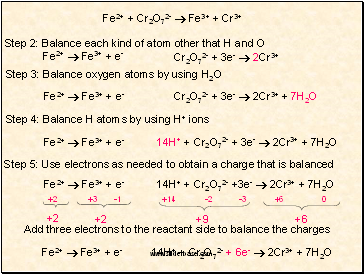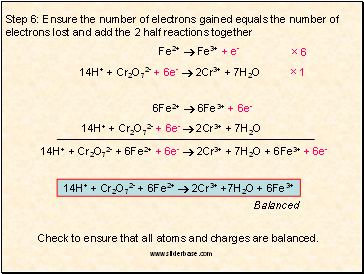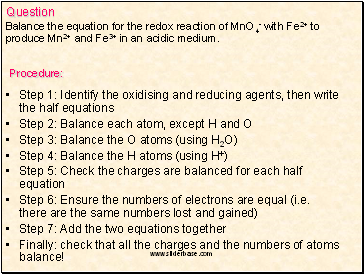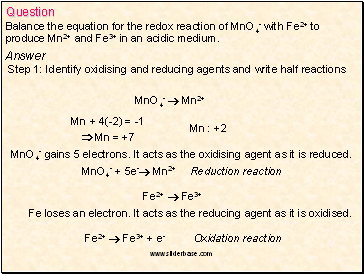# Balancing Chemical Equations. Balancing redox equationsPage 4

#### WATCH ALL SLIDES

Cr26+  Cr3+

Chromium gains three electrons, i.e. it is reduced. It gains its electrons from iron, i.e. it is the oxidising agent.

Oxidation reaction: Fe2+  Fe3+ + e-

Reduction reaction: Cr26+ + 3e-  Cr3+

Half equation:

Half equation:

Slide 15Step 2: Balance each kind of atom other that H and O

Fe2+  Fe3+ + e-

Cr2O72- + 3e-  2Cr3+

Step 3: Balance oxygen atoms by using H2O

Fe2+  Fe3+ + e-

Cr2O72- + 3e-  2Cr3+ + 7H2O

Step 4: Balance H atoms by using H+ ions

Fe2+  Fe3+ + e-

14H+ + Cr2O72- + 3e-  2Cr3+ + 7H2O

Step 5: Use electrons as needed to obtain a charge that is balanced

Fe2+  Fe3+ + e-

14H+ + Cr2O72- +3e-  2Cr3+ + 7H2O

+9

+6

Add three electrons to the reactant side to balance the charges

Fe2+  Fe3+ + e-

14H+ + Cr2O72- + 6e-  2Cr3+ + 7H2O

Fe2+ + Cr2O72-  Fe3+ + Cr3+

+14

-2

-3

+6

0

-1

+3

+2

+2

+2

Slide 16Step 6: Ensure the number of electrons gained equals the number of electrons lost and add the 2 half reactions together

Fe2+  Fe3+ + e-

14H+ + Cr2O72- + 6e-  2Cr3+ + 7H2O

×6

×1

6Fe2+  6Fe3+ + 6e-

14H+ + Cr2O72- + 6e-  2Cr3+ + 7H2O

14H+ + Cr2O72- + 6Fe2+ + 6e-  2Cr3+ + 7H2O + 6Fe3+ + 6e-

14H+ + Cr2O72- + 6Fe2+  2Cr3+ +7H2O + 6Fe3+

Balanced

Check to ensure that all atoms and charges are balanced.

Slide 17Step 1: Identify the oxidising and reducing agents, then write the half equations

Step 2: Balance each atom, except H and O

Step 3: Balance the O atoms (using H2O)

Step 4: Balance the H atoms (using H+)

Step 5: Check the charges are balanced for each half equation

Step 6: Ensure the numbers of electrons are equal (i.e. there are the same numbers lost and gained)

Step 7: Add the two equations together

Finally: check that all the charges and the numbers of atoms balance!

Question

Balance the equation for the redox reaction of MnO4- with Fe2+ to

produce Mn2+ and Fe3+ in an acidic medium.

Procedure:

Slide 18Question

Balance the equation for the redox reaction of MnO4- with Fe2+ to

produce Mn2+ and Fe3+ in an acidic medium.

Step 1: Identify oxidising and reducing agents and write half reactions

MnO4-  Mn2+

Mn + 4(-2) = -1

 Mn = +7

MnO4- gains 5 electrons. It acts as the oxidising agent as it is reduced.

Mn : +2

MnO4- + 5e- Mn2+ Reduction reaction

Go to page:
1  2  3  4  5  6  7  8